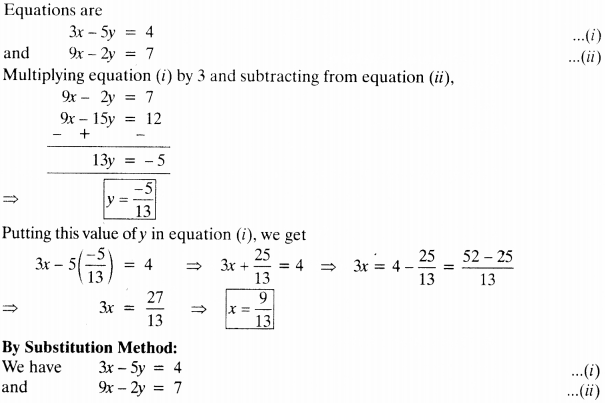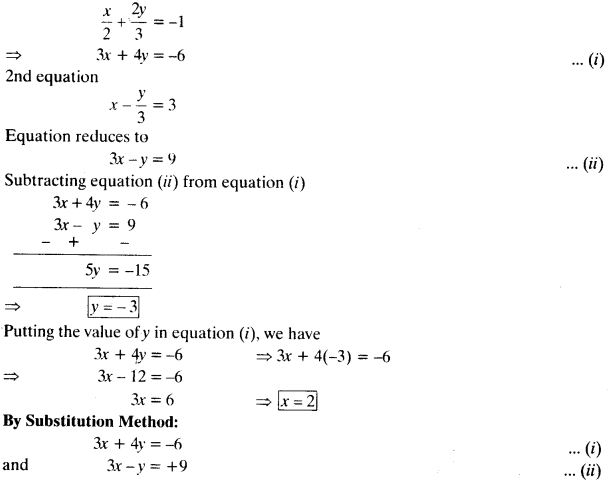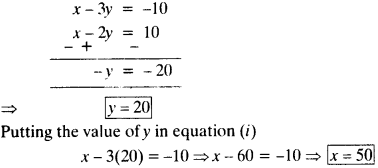# CLASS 10 MATH NCERT SOLUTIONS FOR CHAPTER – 3 PAIR OF LINEAR EQUATIONS IN TWO VARIABLES EX – 3.4

## Pair of Linear Equations in Two Variables

Question 1.
Solve the following pairs of linear equations by the elimination method and the substitution method:Solution:

(i) By Elimination Method:
Fquations are x + y = 5
and 2x – 3y = 4
Multiply equation (i) by 2 and subtract equation (ii) from it, we have(ii)
By Elimination method:
Equations are 3x + 4y = 10
and 2x – 2y = 2
Multiplying equation (ii) by 2 and adding to equation (i), we(iii)
By Elimination Method:(iv) By Elimination Method:
1st equation :Question 2.
Form the pair of linear equations for the following problems and find their solutions (if they exist) by the elimination method:

(i) If we add 1 to the numerator and subtract 1 from the denominator, a fraction reduces to 1. It becomes$\frac { 1 }{ 2 }$, if we only add 1 to the denominator. What is the fraction?

(ii) Five years ago, Nuri was thrice as old as Sonu. Ten years later, Nuri will be twice as old as Sonu. How old are Nuri and Sonu?

(iii) The sum of the digits of a two-digit number is 9. Also, nine times this number is twice the number obtained by reversing the order of the digits. Find the number.

(iv) Meena went to a bank to withdraw ₹2000. She asked the cashier to give her ₹50 and ₹100 notes only. Meena got 25 notes in all. Find how many notes of ₹50 and ₹100 she received.

(v) A lending library has a fixed charge for the first three days and an additional charge for each day thereafter. Saritha paid ₹ 27 for a book kept for seven days, while Susy paid ₹21 for the book she kept for five days. Find the fixed charge and the charge for each extra day.

Solution:

(i) Let numerator be x and denominator be y.
Fraction = x/y
A.T.Q.

(ii)
Let present age of Nuri be x years and Sonu’s present age bey years.
A.T.Q.
1st Condition :

2nd Condition :

Subtractomg equation (ii) from equetion (i), we getHence, present age of Nuri is 50 years and sonu’s present age is 20 years.

(iii) Let digit at unit place = x and digit at ten’s place = y.
Two digit number is lOy + x
A.T.Q.
1st Condition :
x + y = 9
2nd Condition :
9(10y + x) = 2(10k + y) ⇒ 90y + 9x = 20x + 2y
⇒ 88y – 11x = 0 ⇒ -11y + 88y = 0
⇒ -x + 8y = 0
Adding equestion (i) and (ii), we get(iv) Let the number of notes of ₹ 50 = x and the number of notes of ₹ 100 = y
A.T.Q
1st Condition :
50x + 100y = 2000
⇒ x + 2y = 40
2nd Condition :

(v) Let, fixed charge for first 3 days be ₹ x and additional charge per day after 3 days be y.
A.T.Q.
1st Condition : as per Saritha
x + 4y = 27
2nd Condition : as per SusyPutting y = 3 in equation (i),
x + 4(3) = 27 ⇒ x + 12 = 27 ⇒ x = 15
Hence, fixed charge is ₹ 15 and charge for each extra day is ₹ 3.